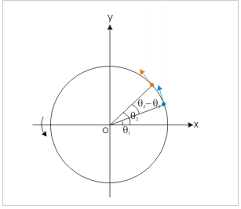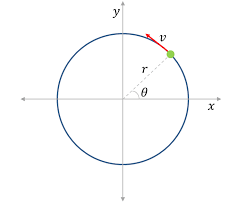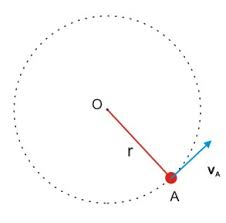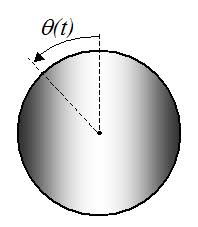## How to Calculate and Solve for Road Bank Angle, Velocity and Radius of a Body in Motion of Circular Path | The Calculator EncyclopediaThe image represents road bank angle in circular motion.

To compute for the road bank angle, three essential parameters are needed and these parameters are velocity (v), acceleration due to gravity (g) and radius (r).

The formula for calculating the road bank angle;

θ = tan-1( / gr)

Where;
v = Velocity
g = Acceleration due to Gravity

Let’s solve an example;
Find the road bank angle where the acceleration due to gravity is 9.8, velocity is 35 and radius is 18.

This implies that;
v = Velocity = 35
g = Acceleration due to Gravity = 9.8

θ = tan-1( / gr)
θ = tan-1(35² / (9.8)(18))
θ = tan-1(1225 / 176.4)
θ = tan-1(6.94)
θ = 81.81°

Therefore, the road bank angle is 81.81°.

Calculating the Velocity when Road Bank Angle, Acceleration due to Gravity and Radius is Given.

v = √gr.tan θ

Where;
v = Velocity
g = Acceleration due to Gravity

Let’s solve an example;
Given that the road bank angle is 50, radius is 15 and acceleration due to gravity is 9.8. Find the velocity?

This implies that;
θ = Road Bank Angle = 50
g = Acceleration due to Gravity = 9.8

v = √gr.tan θ
v = √(9.8 x 15)(tan 50)
v = √(147)(1.1917)
v = √175.1799
v = 13.235

Therefore, the velocity is 13.235.

## How to Calculate and Solve for Centripetal Acceleration, Angular Velocity and Radius of Circular Path of a Body | The Calculator EncyclopediaThe image above represents centripetal acceleration in a motion of circular path.

To compute for the centripetal acceleration, two essential parameters are needed and these parameters are angular velocity (ω) and radius of circular path (r).

The formula for the calculating centripetal acceleration:

a = ω²r

Where:
a = Centripetal Acceleration
ω = Angular Velocity
r = Radius of Circular Path

Let’s solve an example;
Find the centripetal acceleration with an angular velocity of 33 and a radius of 21.

This implies that;
ω = Angular Velocity = 33
r = Radius of Circular Path = 21

a = ω²r
a = 33² x 21
a = 1089 x 21
a = 22869

Therefore, the centripetal acceleration is 22869 m/s².

Calculating the Angular Velocity when Centripetal Acceleration and Radius of Circular path is Given.

ω = √a / r

Where:
ω = Angular Velocity
a = Centripetal Acceleration
r = Radius of Circular Path

Let’s solve an example;
Find the angular velocity with a centripetal acceleration of 320 and a radius of 8.

This implies that;
a = Centripetal Acceleration = 320
r = Radius of Circular Path = 8

ω = √a / r
ω = √320 / 8
ω = √40
ω = 6.32

Therefore, the angular velocity is 6.32.

## How to Calculate and Solve for Maximum Velocity, Coefficient of Friction and Radius of a Body in Motion of Circular Path | Nickzom CalculatorThe image above represents maximum velocity in circular motion.

To compute for the maximum velocity, three essential parameters are needed and these parameters are coefficient of friction (μ), radius (r) and acceleration due to gravity (g).

The formula for calculating maximum velocity:

Vmax = √(μgr)

Where;
Vmax = maximum velocity
μ = coefficient of friction
g = acceleration due to gravity

Let’s solve an example;
Find the maximum velocity when the coefficient of friction is 14 with a radius of 7 and acceleration due to gravity of 9.8.

This implies that;
μ = coefficient of friction = 14
g = acceleration due to gravity = 9.8

Vmax = √(μgr)
Vmax = √(14 x 7 x 9.8)
Vmax = √(960.40)
Vmax = 30.99

Therefore, the maximum velocity is 30.99 m/s.

Calculating the Coefficient of Friction when the Maximum Velocity, Radius and Acceleration due to Gravity is Given.

μ = Vmax2 / gr

Where;
μ = coefficient of friction
Vmax = maximum velocity
g = acceleration due to gravity

Let’s solve an example;
Find the coefficient of friction with a maximum velocity of 120, radius of 15 and acceleration due to gravity is 9.8?

This implies that;
Vmax = maximum velocity = 120
g = acceleration due to gravity = 9.8

μ = Vmax2 / gr
μ = 1202 / 15 x 9.8
μ = 14400 / 147
μ = 97.96

Therefore, the coefficient of friction is 97.96.

## How to Calculate and Solve for Superelevation, Guage of Track, Velocity and Radius of a Body in Circular Path Motion | The Calculator EncyclopediaThe image above represents a body in superelevation.

To compute for the Superelevation, four essential parameters are needed and these parameters are Gauge of the track (G), velocity of the body (v), radius of the curve (r) and acceleration due to gravity (g).

The formula for calculating the circular path motion:

S = Gv² / gr

Where:
S = Superelevation
G = Gauge of the track
v = velocity of the body
r = radius of the curve
g = acceleration due to gravity

Let’s solve an example;
Find the circular path motion when Gauge of the track is 14, velocity of the track is 47, radius of the curve is 21 and acceleration due to gravity is 9.8.

This implies that;
G = Gauge of the track = 14
v = velocity of the body = 47
r = radius of the curve = 21
g = acceleration due to gravity = 9.8

S = Gv² / gr
S = 14 x 47² / 9.8 x 21
S = 14 x 2209 / 205.8
S = 30926 / 205.8
S = 150.27

Therefore, the superelevation is 150.27 m.

Calculating the Gauge of the track when Superelevation, Velocity of the body, Radius of the curve and Acceleration due to gravity.

G = Sgr / v2

Where;
G = Gauge of the track
S = Superelevation
v = velocity of the body
r = radius of the curve
g = acceleration due to gravity

Let’s solve an example;
With a superelevation of 180, velocity of the body is 32, radius of the curve is 12 and acceleration due to gravity as 9.8. Find the gauge of the track?

This implies that;
S = Superelevation = 180
v = velocity of the body = 32
r = radius of the curve = 12
g = acceleration due to gravity = 9.8

G = Sgr / v2
G = 180 x 12 x 9.8 / 322
G = 21168 / 1024
G = 20.67

Therefore, the gauge of the track is 20.67.

Calculating the Velocity of the body when Superelevation, Gauge of the track, Radius of the curve and Acceleration due to gravity.

v = √Sgr / G

Where;
v = velocity of the body
S = Superelevation
G = Gauge of the track
r = radius of the curve
g = acceleration due to gravity

Let’s solve an example;
With a superelevation of 120, gauge of the track is 28, radius of the curve is 7 and acceleration due to gravity as 9.8. Find the velocity of the body?

This implies that;
S = Superelevation = 120
G = gauge of the track = 28
r = radius of the curve = 7
g = acceleration due to gravity = 9.8

v = √Sgr / G
v = √120 x 9.8 x 7 / 28
v = √8232 / 28
v = √294
v = 17.146

Therefore, the velocity of the body is 17.146.